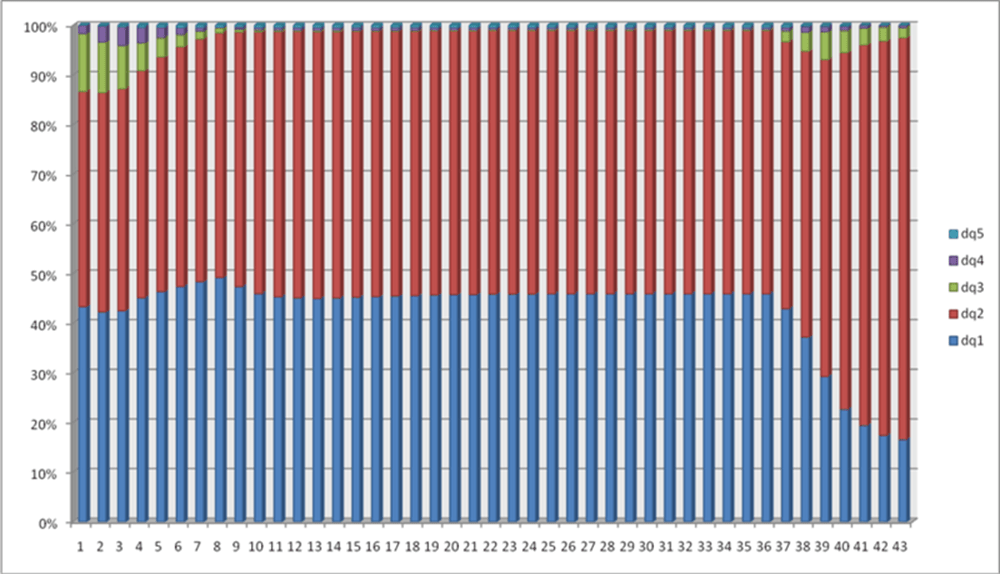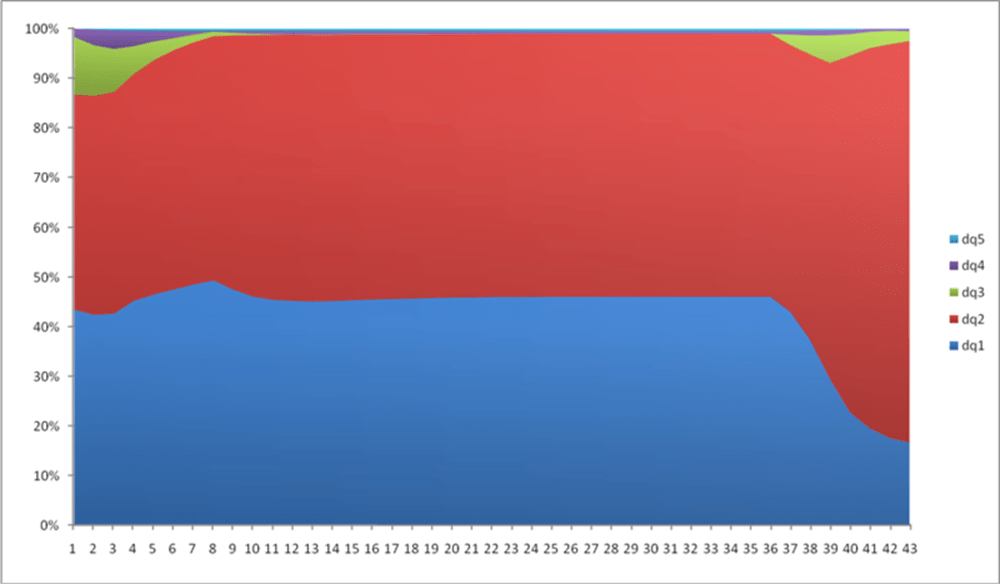# What are the Units for the Six St. Venant Flow Terms in SWMM 5 and InfoSWMM?

## What are the Units for the Six St. Venant Flow Terms in SWMM 5 and InfoSWMM?

Subject: What are the Units for the six St. Venant Flow Terms in SWMM 5 and InfoSWMM?

The new flow (Q) calculated at during each iteration of time step as

(1) Q for the new iteration = (Q at the Old Time Step – DQ2 + DQ3 + DQ4 ) / ( 1.0 + DQ1 + DQ5)

In which DQ2, DQ3 and DQ4 all have units of flow (note internally SWMM 5 has units of CFS and the flows are converted to the user units in the output file, graphs and tables of SWMM 5).

The equations and units for DQ2, DQ3 and DQ4 are:

(2) Units of DQ2 = DT * GRAVITY * aWtd * ( H2 – H1) / Length = second * feet/second^2 * feet^2 * feet / feet = feet^3/second = CFS

(3) Units of DQ3 = 2 * Velocity * ( aMid – aOld) * Sigma = feet/second * feet^2 = feet^3/second = CFS

(4) Units of DQ4 = DT * Velocity * Velocity * ( aMid – aOld) * Sigma / Length = second * feet/second * feet/second * feet^2 / feet = feet^3/second = CFS

The equations and units for DQ1 and DQ5 are:

(5) Units of DQ1 = DT * GRAVITY * (n/PHI)^2 * Velocity / Hydraulic Radius^1.333 = second * feet/second^2 * second^2 * feet^1/3 * feet/second / feet^1.33 = Dimensionless

(6) Units of DQ5 = K * Q / Area / 2 / Length * DT = feet^3/second * 1/feet^2 * 1/feet * second = Dimensionless

The five components calculated at the each time step and at each iteration during a time step and together predict the new Link Flow (Q) in SWMM 5. The value of the different components can be seen over time in Figure 1 and as a component percentage in Figure 2 and 3.Figure 1: The Six St. Venant Components over time.Figure 2: The relative magnitude of the St Venant terms over time for the same for the same link as in Figure 1.Figure 3: The relative magnitude of the St Venant terms over time for the same for the same link as in Figure 1 shown in an area chart normalized to 100 percent. Normally the DQ1 and DQ2 terms balance each other except for backwater conditions or reverse flow in which the terms DQ3 and DQ4 can dominate.

Translate »